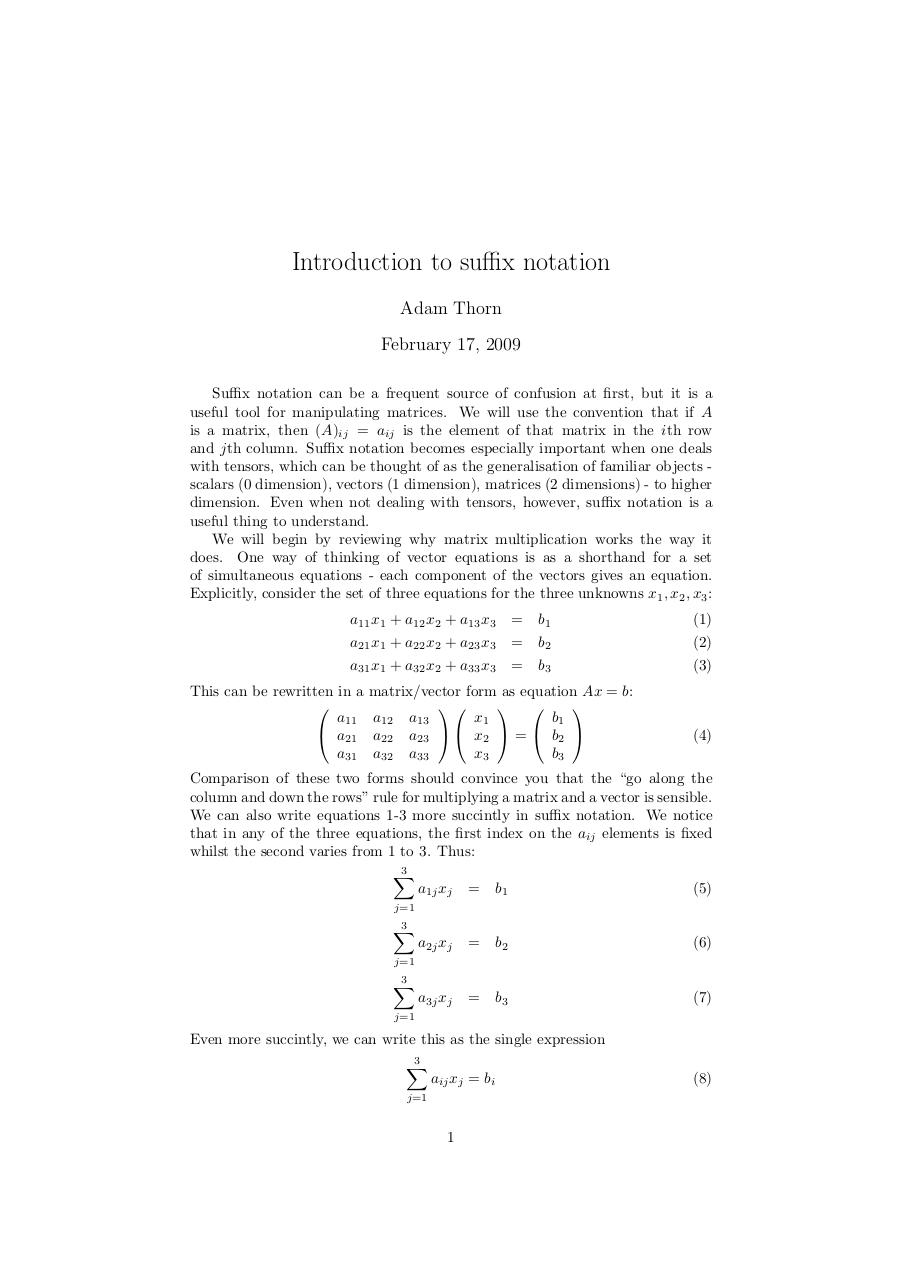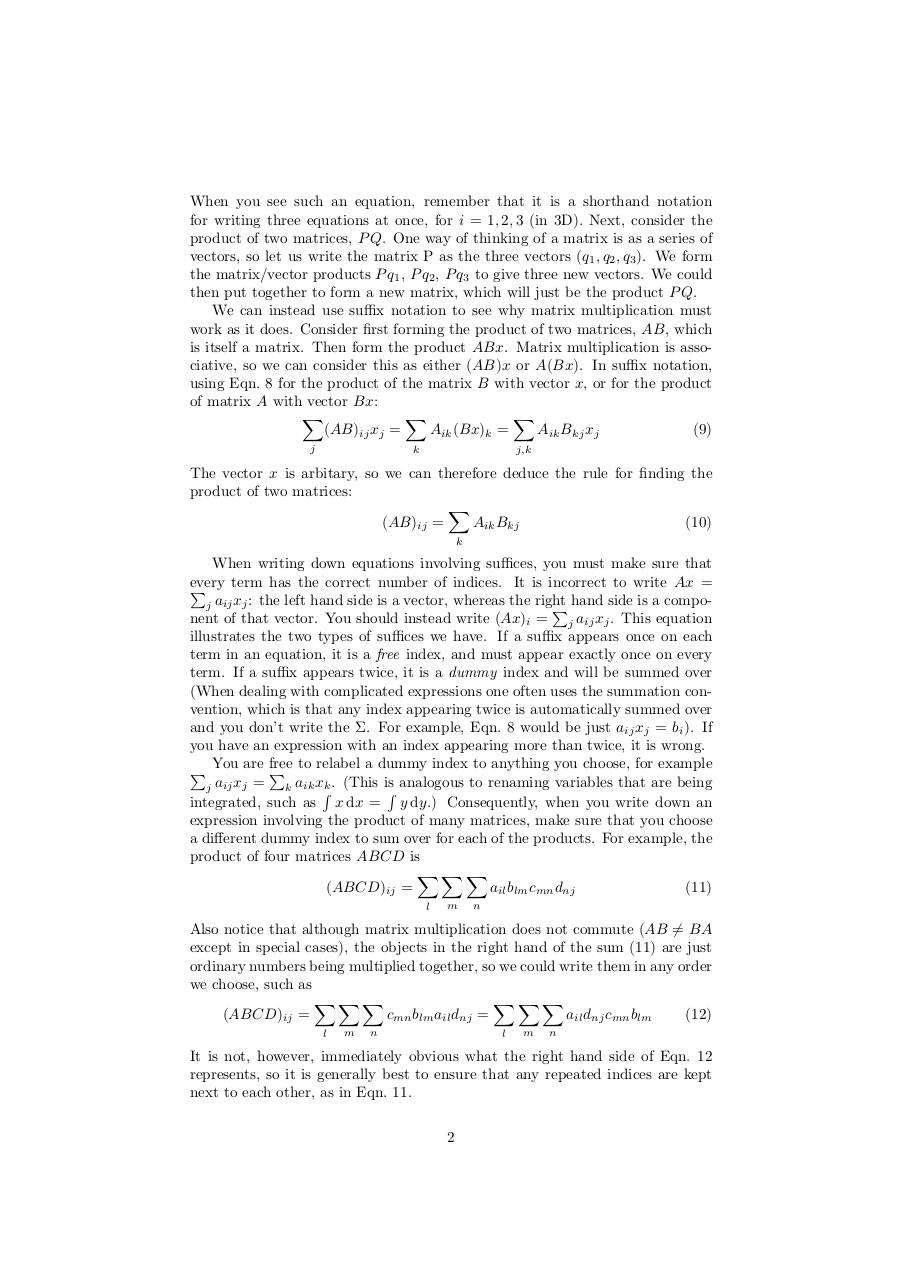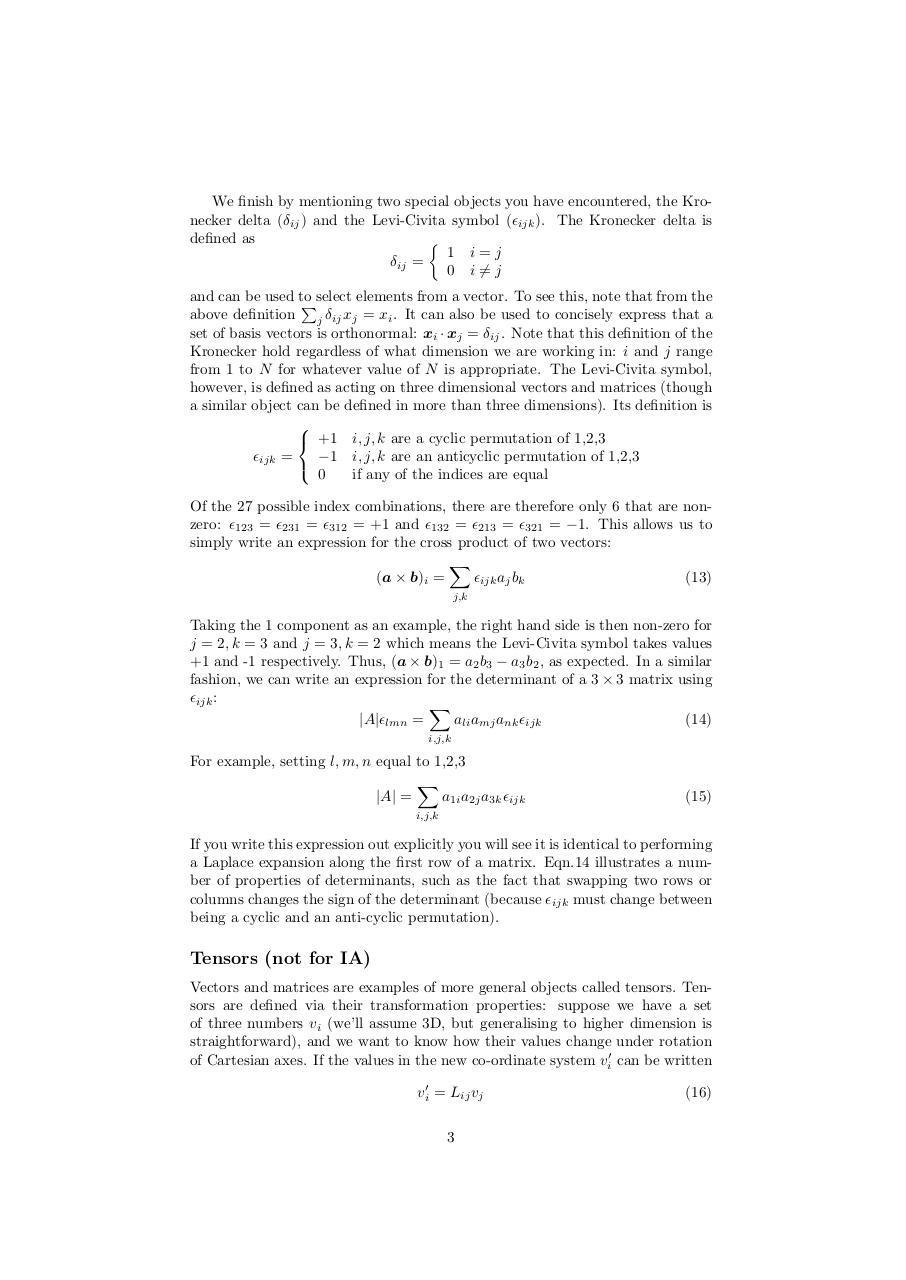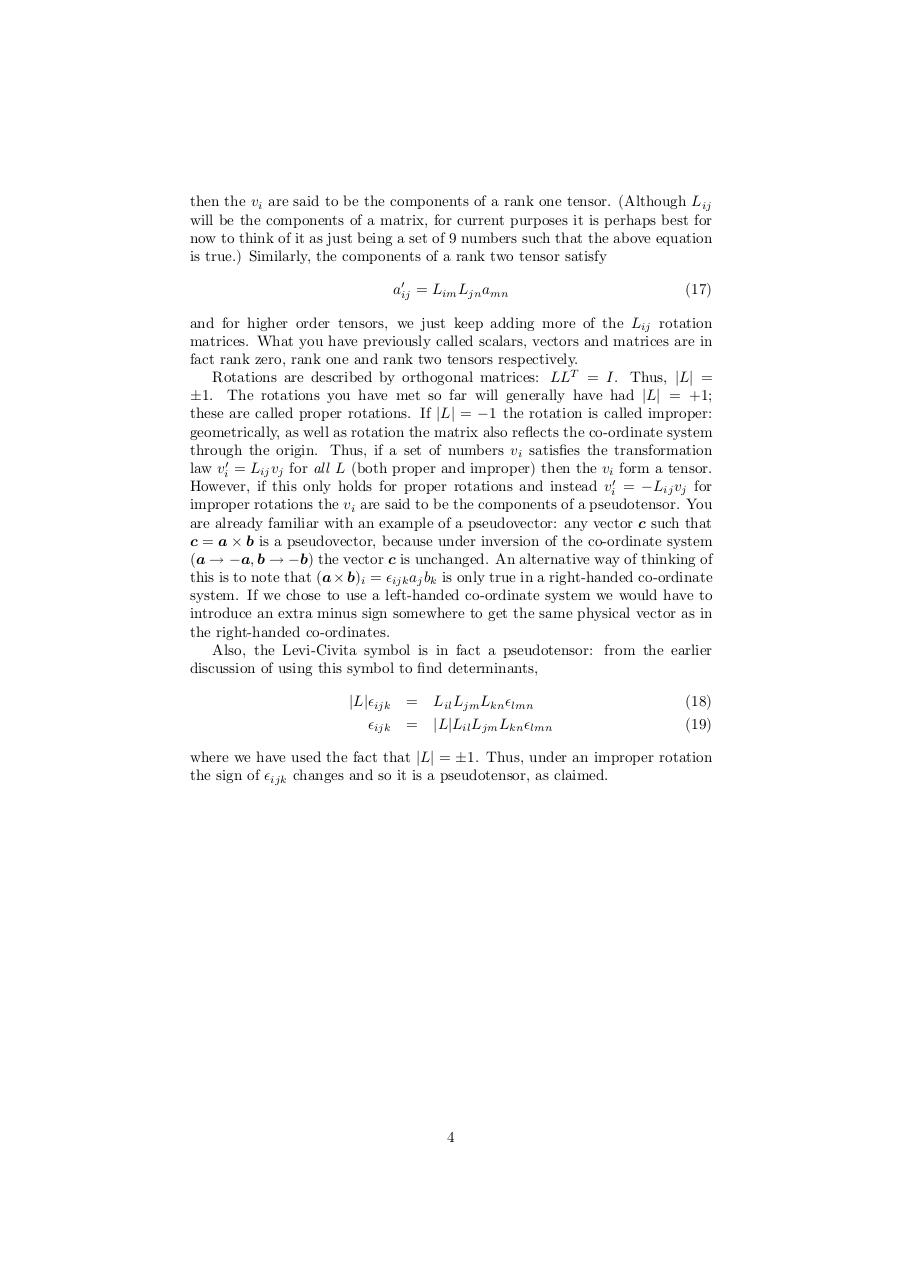# suffices .pdf

### File information

Original filename: suffices.pdf

This PDF 1.4 document has been generated by TeX / pdfeTeX-1.21a, and has been sent on pdf-archive.com on 06/05/2014 at 16:07, from IP address 131.111.x.x. The current document download page has been viewed 1406 times.
File size: 77 KB (4 pages).
Privacy: public file

suffices.pdf (PDF, 77 KB)

### Document preview

Introduction to suffix notation
February 17, 2009
Suffix notation can be a frequent source of confusion at first, but it is a
useful tool for manipulating matrices. We will use the convention that if A
is a matrix, then (A)ij = aij is the element of that matrix in the ith row
and jth column. Suffix notation becomes especially important when one deals
with tensors, which can be thought of as the generalisation of familiar objects scalars (0 dimension), vectors (1 dimension), matrices (2 dimensions) - to higher
dimension. Even when not dealing with tensors, however, suffix notation is a
useful thing to understand.
We will begin by reviewing why matrix multiplication works the way it
does. One way of thinking of vector equations is as a shorthand for a set
of simultaneous equations - each component of the vectors gives an equation.
Explicitly, consider the set of three equations for the three unknowns x1 , x2 , x3 :
a11 x1 + a12 x2 + a13 x3

= b1

(1)

a21 x1 + a22 x2 + a23 x3

= b2

(2)

a31 x1 + a32 x2 + a33 x3

= b3

(3)

This can be rewritten in a

a11
 a21
a31

matrix/vector form as equation Ax = b:

 

a12 a13
b1
x1
a22 a23   x2  =  b2 
b3
x3
a32 a33

(4)

Comparison of these two forms should convince you that the “go along the
column and down the rows” rule for multiplying a matrix and a vector is sensible.
We can also write equations 1-3 more succintly in suffix notation. We notice
that in any of the three equations, the first index on the aij elements is fixed
whilst the second varies from 1 to 3. Thus:
3
X
a1j xj = b1
(5)
j=1
3
X

a2j xj

= b2

(6)

a3j xj

= b3

(7)

j=1
3
X
j=1

Even more succintly, we can write this as the single expression
3
X

aij xj = bi

j=1

1

(8)

When you see such an equation, remember that it is a shorthand notation
for writing three equations at once, for i = 1, 2, 3 (in 3D). Next, consider the
product of two matrices, P Q. One way of thinking of a matrix is as a series of
vectors, so let us write the matrix P as the three vectors (q1 , q2 , q3 ). We form
the matrix/vector products P q1 , P q2 , P q3 to give three new vectors. We could
then put together to form a new matrix, which will just be the product P Q.
We can instead use suffix notation to see why matrix multiplication must
work as it does. Consider first forming the product of two matrices, AB, which
is itself a matrix. Then form the product ABx. Matrix multiplication is associative, so we can consider this as either (AB)x or A(Bx). In suffix notation,
using Eqn. 8 for the product of the matrix B with vector x, or for the product
of matrix A with vector Bx:
X
X
X
(AB)ij xj =
Aik (Bx)k =
Aik Bkj xj
(9)
j

k

j,k

The vector x is arbitary, so we can therefore deduce the rule for finding the
product of two matrices:
X
(AB)ij =
Aik Bkj
(10)
k

When writing down equations involving suffices, you must make sure that
every
term has the correct number of indices. It is incorrect to write Ax =
P
a
xj : the left hand side is a vector, whereas the right
ij
j
P hand side is a component of that vector. You should instead write (Ax)i = j aij xj . This equation
illustrates the two types of suffices we have. If a suffix appears once on each
term in an equation, it is a free index, and must appear exactly once on every
term. If a suffix appears twice, it is a dummy index and will be summed over
(When dealing with complicated expressions one often uses the summation convention, which is that any index appearing twice is automatically summed over
and you don’t write the Σ. For example, Eqn. 8 would be just aij xj = bi ). If
you have an expression with an index appearing more than twice, it is wrong.
P You are free
P to relabel a dummy index to anything you choose, for example
a
x
=
ij
j
k . (This is analogous to renaming variables that are being
j
k aik xR
R
integrated, such as x dx = y dy.) Consequently, when you write down an
expression involving the product of many matrices, make sure that you choose
a different dummy index to sum over for each of the products. For example, the
product of four matrices ABCD is
XXX
(ABCD)ij =
ail blm cmn dnj
(11)
l

m

n

Also notice that although matrix multiplication does not commute (AB 6= BA
except in special cases), the objects in the right hand of the sum (11) are just
ordinary numbers being multiplied together, so we could write them in any order
we choose, such as
XXX
XXX
(ABCD)ij =
cmn blm ail dnj =
ail dnj cmn blm
(12)
l

m

n

l

m

n

It is not, however, immediately obvious what the right hand side of Eqn. 12
represents, so it is generally best to ensure that any repeated indices are kept
next to each other, as in Eqn. 11.
2

We finish by mentioning two special objects you have encountered, the Kronecker delta (δij ) and the Levi-Civita symbol (ijk ). The Kronecker delta is
defined as

1 i=j
δij =
0 i 6= j
and can be used to
Pselect elements from a vector. To see this, note that from the
above definition j δij xj = xi . It can also be used to concisely express that a
set of basis vectors is orthonormal: xi · xj = δij . Note that this definition of the
Kronecker hold regardless of what dimension we are working in: i and j range
from 1 to N for whatever value of N is appropriate. The Levi-Civita symbol,
however, is defined as acting on three dimensional vectors and matrices (though
a similar object can be defined in more than three dimensions). Its definition is

 +1 i, j, k are a cyclic permutation of 1,2,3
−1 i, j, k are an anticyclic permutation of 1,2,3
ijk =

0
if any of the indices are equal
Of the 27 possible index combinations, there are therefore only 6 that are nonzero: 123 = 231 = 312 = +1 and 132 = 213 = 321 = −1. This allows us to
simply write an expression for the cross product of two vectors:
X
(a × b)i =
ijk aj bk
(13)
j,k

Taking the 1 component as an example, the right hand side is then non-zero for
j = 2, k = 3 and j = 3, k = 2 which means the Levi-Civita symbol takes values
+1 and -1 respectively. Thus, (a × b)1 = a2 b3 − a3 b2 , as expected. In a similar
fashion, we can write an expression for the determinant of a 3 × 3 matrix using
ijk :
X
|A|lmn =
ali amj ank ijk
(14)
i,j,k

For example, setting l, m, n equal to 1,2,3
X
|A| =
a1i a2j a3k ijk

(15)

i,j,k

If you write this expression out explicitly you will see it is identical to performing
a Laplace expansion along the first row of a matrix. Eqn.14 illustrates a number of properties of determinants, such as the fact that swapping two rows or
columns changes the sign of the determinant (because ijk must change between
being a cyclic and an anti-cyclic permutation).

Tensors (not for IA)
Vectors and matrices are examples of more general objects called tensors. Tensors are defined via their transformation properties: suppose we have a set
of three numbers vi (we’ll assume 3D, but generalising to higher dimension is
straightforward), and we want to know how their values change under rotation
of Cartesian axes. If the values in the new co-ordinate system vi0 can be written
vi0 = Lij vj
3

(16)

then the vi are said to be the components of a rank one tensor. (Although Lij
will be the components of a matrix, for current purposes it is perhaps best for
now to think of it as just being a set of 9 numbers such that the above equation
is true.) Similarly, the components of a rank two tensor satisfy
a0ij = Lim Ljn amn

(17)

and for higher order tensors, we just keep adding more of the Lij rotation
matrices. What you have previously called scalars, vectors and matrices are in
fact rank zero, rank one and rank two tensors respectively.
Rotations are described by orthogonal matrices: LLT = I. Thus, |L| =
±1. The rotations you have met so far will generally have had |L| = +1;
these are called proper rotations. If |L| = −1 the rotation is called improper:
geometrically, as well as rotation the matrix also reflects the co-ordinate system
through the origin. Thus, if a set of numbers vi satisfies the transformation
law vi0 = Lij vj for all L (both proper and improper) then the vi form a tensor.
However, if this only holds for proper rotations and instead vi0 = −Lij vj for
improper rotations the vi are said to be the components of a pseudotensor. You
are already familiar with an example of a pseudovector: any vector c such that
c = a × b is a pseudovector, because under inversion of the co-ordinate system
(a → −a, b → −b) the vector c is unchanged. An alternative way of thinking of
this is to note that (a × b)i = ijk aj bk is only true in a right-handed co-ordinate
system. If we chose to use a left-handed co-ordinate system we would have to
introduce an extra minus sign somewhere to get the same physical vector as in
the right-handed co-ordinates.
Also, the Levi-Civita symbol is in fact a pseudotensor: from the earlier
discussion of using this symbol to find determinants,
|L|ijk
ijk

= Lil Ljm Lkn lmn

(18)

= |L|Lil Ljm Lkn lmn

(19)

where we have used the fact that |L| = ±1. Thus, under an improper rotation
the sign of ijk changes and so it is a pseudotensor, as claimed.

4#### HTML Code

Copy the following HTML code to share your document on a Website or Blog

#### QR Code### Related keywords